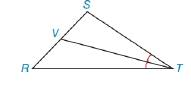Chapter 8.1, Problem 54E### Elementary Geometry for College St...

6th Edition
Daniel C. Alexander + 1 other
ISBN: 9781285195698

#### Solutions

Chapter
Section### Elementary Geometry for College St...

6th Edition
Daniel C. Alexander + 1 other
ISBN: 9781285195698
Textbook Problem
1 views

# T V ¯ bisects ∠ S T R of △ S T R . S T = 6 and T R = 9. If the area of △ S T R is 25  m 2 , find the area of △ S V T .To determine

To find:

The area of ΔSVT.

Explanation

“A median of a triangle separate it into two triangle of equal area.”

Calculation:

Given:

TV¯ bisects STR of ΔSTR.

ST=6 m

RT=9 m

ASTR=25 m2

TV¯ is bisect, the angle of ΔSTR.

(i.e.) TV¯ is the median of the line SR.

Since, A median of a triangle separate it into two triangle of equal area.

Let A1 and A2 are the areas of the triangle ΔSVT and ΔRVT.

Here, TV¯ bisects the ΔSTR into the two equal areas of triangles ΔSVT and ΔRVT

### Still sussing out bartleby?

Check out a sample textbook solution.

See a sample solution

#### The Solution to Your Study Problems

Bartleby provides explanations to thousands of textbook problems written by our experts, many with advanced degrees!

Get Started

#### Find the domain of the function. 37. F(p)=2p

Single Variable Calculus: Early Transcendentals

#### The graph at the right has equation:

Study Guide for Stewart's Multivariable Calculus, 8th

#### True or False: f(x) = 4x2 8x + 1 is increasing on (0, 1).

Study Guide for Stewart's Single Variable Calculus: Early Transcendentals, 8th